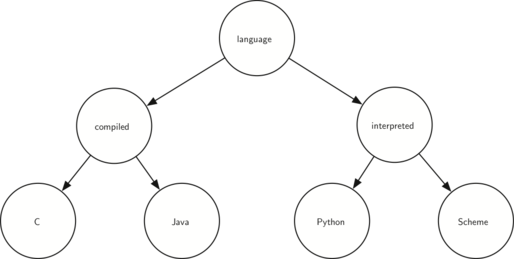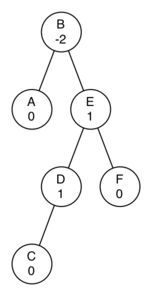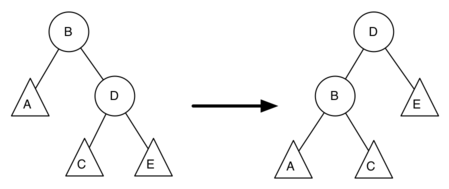# 8.20. Discussion Questions¶

1. Draw the tree structure resulting from the following set of tree function calls:

>>> r = BinaryTree(3)
>>> insertLeft(r,4)
[3, [4, [], []], []]
>>> insertLeft(r,5)
[3, [5, [4, [], []], []], []]
>>> insertRight(r,6)
[3, [5, [4, [], []], []], [6, [], []]]
>>> insertRight(r,7)
[3, [5, [4, [], []], []], [7, [], [6, [], []]]]
>>> setRootVal(r,9)
>>> insertLeft(r,11)
[9, [11, [5, [4, [], []], []], []], [7, [], [6, [], []]]]

2. Trace the algorithm for creating an expression tree for the expression $$(4 * 8) / 6 - 3$$.

3. Consider the following array of integers: [1,2,3,4,5,6,7,8,9,10]. Show the binary search tree resulting from inserting the integers in the array.

4. Consider the following array of integers: [10,9,8,7,6,5,4,3,2,1]. Show the binary search tree resulting from inserting the integers in the array.

5. Generate a random array of integers. Show the binary heap tree resulting from inserting the integers on the array one at a time.

6. Using the array from the previous question, show the binary heap tree resulting from using the array as a parameter to the buildHeap method. Show both the tree and array form.

7. Draw the binary search tree that results from inserting the following keys in the order given: 68,88,61,89,94,50,4,76,66, and 82.

8. Generate a random array of integers. Draw the binary search tree resulting from inserting the integers on the array.

9. Consider the following array of integers: [1,2,3,4,5,6,7,8,9,10]. Show the binary heap resulting from inserting the integers one at a time.

10. Consider the following array of integers: [10,9,8,7,6,5,4,3,2,1]. Show the binary heap resulting from inserting the integers one at a time.

11. Consider the two different techniques we used for implementing traversals of a binary tree. Why must we check before the call to preorder when implementing as a method, whereas we could check inside the call when implementing as a function?

1. Show the function calls needed to build the following binary tree.1. Given the following tree, perform the appropriate rotations to bring it back into balance.1. Using the following as a starting point, derive the equation that gives the updated balance factor for node D.